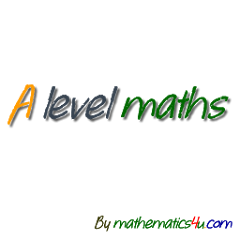# A Level Maths - Advanced Level

10K+Everyone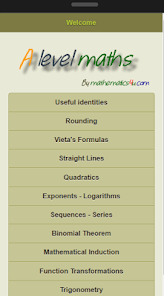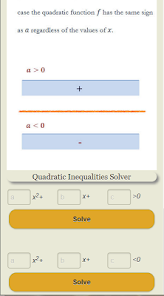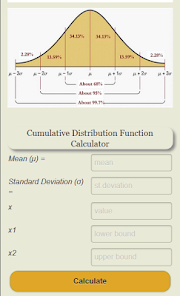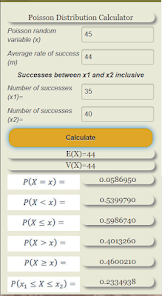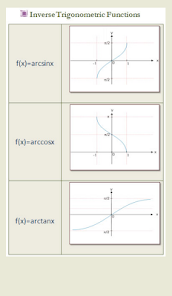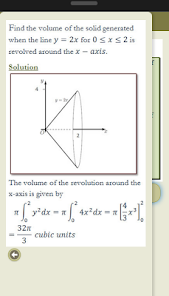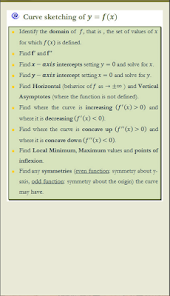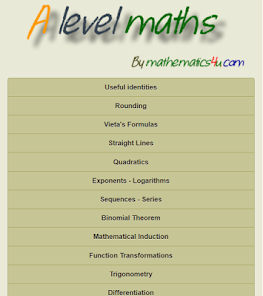An "A level Maths" revision guide app, with video lessons, many calculators, solvers and it’s 100% free, No in-app purchases, No advertisements. A revision guide app for A Level Mathematics and other math educational programs of the same level as well with many solvers and Video Lessons. This app intends to help students understand the main math topics of their syllabuses.This app has been developed independently of any educational organization and is in no way endorsed by any educational organization.

The topics covered in this version are the following:

Useful Identities
Rounding in Significant Figures with a Calculator
Numerical Methods
Vieta's Formulas
Straight Lines
Parametric Equations
Exponents and Logarithms
Exponential and Logarithmic Functions
Arithmetic Sequences and Series and an Arithmetic Sequence Calculator which is used to calculate the nth term and the sum of the first n terms of arithmetic sequence.
Geometric Sequences and Series and an Geometric Sequence Calculator.
Series - Sigma notation
Compound Interest Calculator.
Binomial Theorem
Mathematical Induction
Function Transformations (Horizontal Translation, Vertical Translation, Horizontal Stretch - Shrink, Vertical Stretch - Shrink, Reflections, Absolute value Transformations)

Trigonometry (Trigonometric Equations, Trigonometric Functions,Trigonometric Identities, etc)

Differentiation (Derivative, Differentiation Rules, Derivative Tables, Implicit Differentiation, Tangents & Normals, Stationary Points, Points of Inflexion, Curve Sketching, Graphs of f(x) and its derivative )

Integration (Indefinite Integral, Definite Integral, Integral Table, Integration Techniques)

Applications of Integration (Area between a curve and x-axis, Area between a curve and y-axis, Area between two curves, Volume of revolution, Kinematics)

Complex Numbers (Operations with Complex Numbers, Complex Conjugates, Equations, Cartesian form, Polar form, Exponential Form, Argand Diagram, Modulus and Argument, De Moivre's Theorem, Roots of Complex Numbers)

Matrix Algebra (Matrices. Determinants, Linear Transformations)

Vectors, Lines, Planes
Vector & Scalar Projection with a Calculator
Dot Product with a Calculator
Cross Product with a Calculator

Sets and Probability (Sets, Probability, Conditional Probability, Independent events, Bayes' theorem)
Combination, Permutation and Arrangements Calculator.
Conditional Calculator computes the probability of event A occurring, given that event B has occurred and vice versa.
Normal Distribution Calculator.
Binomial Distribution Calculator.
Poisson Distribution Calculator.
and finally a Revision Quiz which requires an internet connection.

We provide updates periodically to improve the performance and the functionality of the app. You should install the latest version of the app for the best experience.

The information provided on this app is for educational purposes and its accuracy is not guaranteed.The content of this app has been researched and published with the utmost care and attention, however errors in the process cannot be ruled out. Please send any comments and corrections to:
apps@ibmaths4u.com
In spite of the most careful checking, we cannot accept liability for the correctness, completeness and up-to-dateness of this app. We do not accept liability in particular for any damage or consequences caused by direct or indirect use of the content of the app.The revision material may not cover all areas necessary for any particular examination or examination body.
Content contained (apart from the video lessons) on this app is intellectual property of ©ibmaths4u.com and may not be copied, reproduced, distributed or displayed without ©ibmaths4u.com ‘s express written permission.
Updated on
Oct 27, 2020

## Data safety